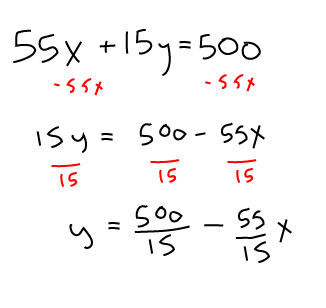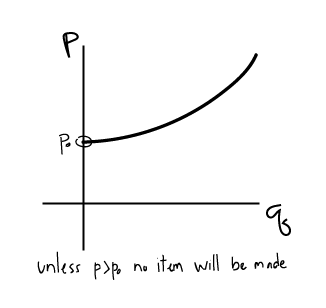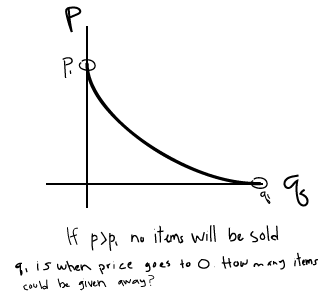# Bloomington Tutors Blog Finite math, K201, calculus, and statistics explained

## A linear programming word problem - with a surprise twist!

Finite M118

Today, I thought I'd tackle a problem from one of the most "popular" courses (read "required for almost all majors") at this school - finite math. For those of you who haven't heard of this course before, finite math is actually an amalgam of miscellaneous topics from probability theory, linear algebra, and stats, created by math departments at various universities as a way to introduce college freshmen to rigorous, analytical thinking. It's not a real field of mathematics, in the sense that you won't find any professional mathematicians who specialize in "finite mathematics." However, there are mathematicians who specialize in the topics introduced in this course, such as combinatorics and probability.

One of the topics covered in finite math ("finite", by those in the know) is linear programming. This is basically a fancy term for a constrained optimization problem consisting of linear constraints and a linear objective function. In this blog post, I will tackle the followin...

Continue Reading...

Calculus M119

## 1.4b Budget constraint problem with fixed prices for 2 items

You have a budget of 500 dollars for books ($x$) and CDs ($y$). The total spent on books is $55x$ and the total spent on CDs is $15y$. Write an equation in the form of $y = mx+b$ then solve for $y$.## Supply and demand problems

Supply and demand both relate quantity $q$ of an item to the price $p$.

### Supply curve: Increasing function. $q$ increases as $p$ increases

• The higher the price that sellers can charge for an item, the more quantity they will produce.
• If sellers can NOT sell an item for more money than it costs to manufacture, then they would choose not to manufacture it.### Demand Curve: Decreasing function. $q$ decreases as $p$ increases

• The more an item costs, the less buyers will want to buy it (less quantity will be demanded).
• If price is too high, NO items will be sold.### Equilibrium point: It is assumed that the market settles to an equilibrium point when $S(p) = D(p)$...

Continue Reading...

## Practice for your finite exams with our sample problems!

Finite M118

Welcome, new and returning IU students! I hope everyone had a great summer and is ready (sorta?) to get back to class. Here at Bloomington Tutors, we've been working hard all summer and getting ready to help you succeed.

We've launched our practice exam system for finite math (M118), and hope to start adding materials for calculus and other courses soon as well. Check out our finite questions here: https://bloomingtontutors.com/quiz/finite-math

They're interactive multiple-choice questions, with explanations for the wrong answers (i.e., we try to guess the common mistakes you might have made to get that answer, and offer you an explanation).

Enjoy!